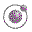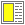Higher-order Functions - slide 30 : 34

Tables with higher-order functions
Instead of explicit composition of td and tr elements we can use a mapping to apply tr to rows and td to elements
Expression

Value

```(define rows
'(("This" "is" "first" "row")
("This" "is" "second" "row")
("This" "is" "third" "row")
("This" "is" "fourth" "row"))
)

(table 'border 5
(gmap
(compose tr (gmap td)) rows))```
 This is first row This is second row This is third row This is fourth row

The last example illustrates that (gmap td) is a useful building block, which can be composed with other functions.

The last example depends on the fact that the HTML mirror functions accept lists of elements and attributes.